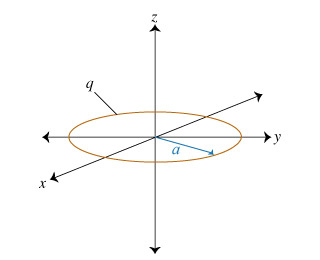# Problem: Consider a uniformly charged ring in the xy plane, centered at the origin. The ring has radius a and positive charge q distributed evenly along its circumference. What is the magnitude of the electric field along the positive z axis?Use k in your answer where:k=14πε0E(x) = ______

###### FREE Expert Solution

Electric filed:

$\overline{){\mathbf{E}}{\mathbf{=}}\frac{\mathbf{k}\mathbf{q}}{{\mathbf{r}}^{\mathbf{2}}}}$

Consider a point with the point z, located on the positive z-axis. The ring has a radius a. An electric field vector originating from an element of charge on the ring makes an angle θ with the z-axis.

The adjacent side has a length z, the opposite side has a length a and the hypotenuse is equal to (z2 + a2)(1/2). The net electric field is directed along the z-axis.

80% (115 ratings)###### Problem Details

Consider a uniformly charged ring in the xy plane, centered at the origin. The ring has radius a and positive charge q distributed evenly along its circumference.What is the magnitude of the electric field along the positive z axis?

$\mathbf{k}\mathbf{=}\frac{\mathbf{1}}{\mathbf{4}{\mathbf{\pi \epsilon }}_{\mathbf{0}}}$